# RD Sharma Solutions for Class 11 Chapter 19 - Arithmetic Progressions Exercise 19.5

In this exercise, students will be solving problems based on the properties of arithmetic progressions. For students who find difficulty in solving problems, the RD Sharma Solutions for Class 11 Maths is the right study material one can opt for. Students can quickly refer to RD Sharma solutions for any doubts pertaining to the problems. Experts at BYJU’S have created the solutions as per the guidelines, in accordance with the latest CBSE patterns, which are easily available in pdf format too. Students can refer to RD Sharma Class 11 Maths Solutions pdf and can download them, which is available in the links attached below.

## Download the pdf of RD Sharma Solutions for Class 11 Maths Exercise 19.5 Chapter 19 – Arithmetic Progressions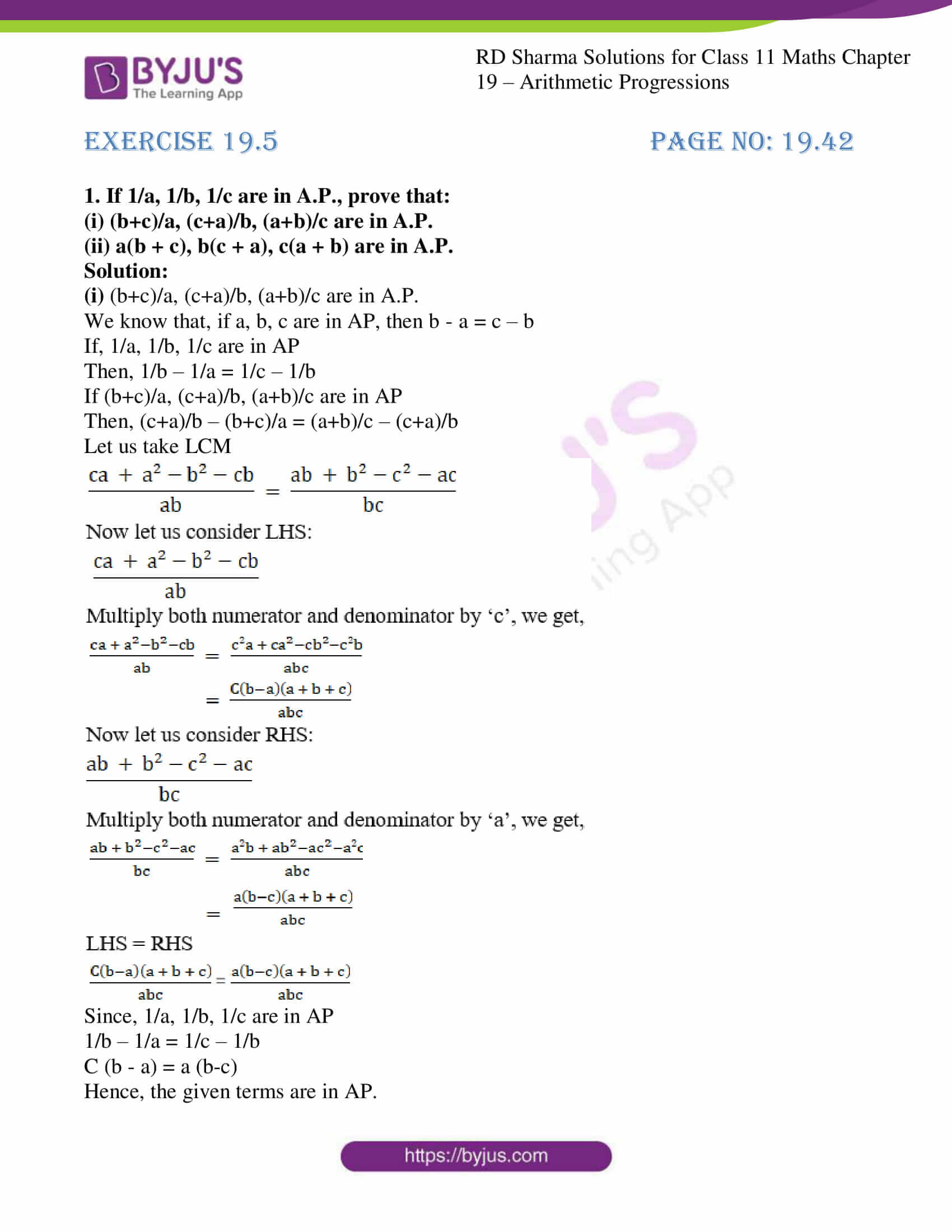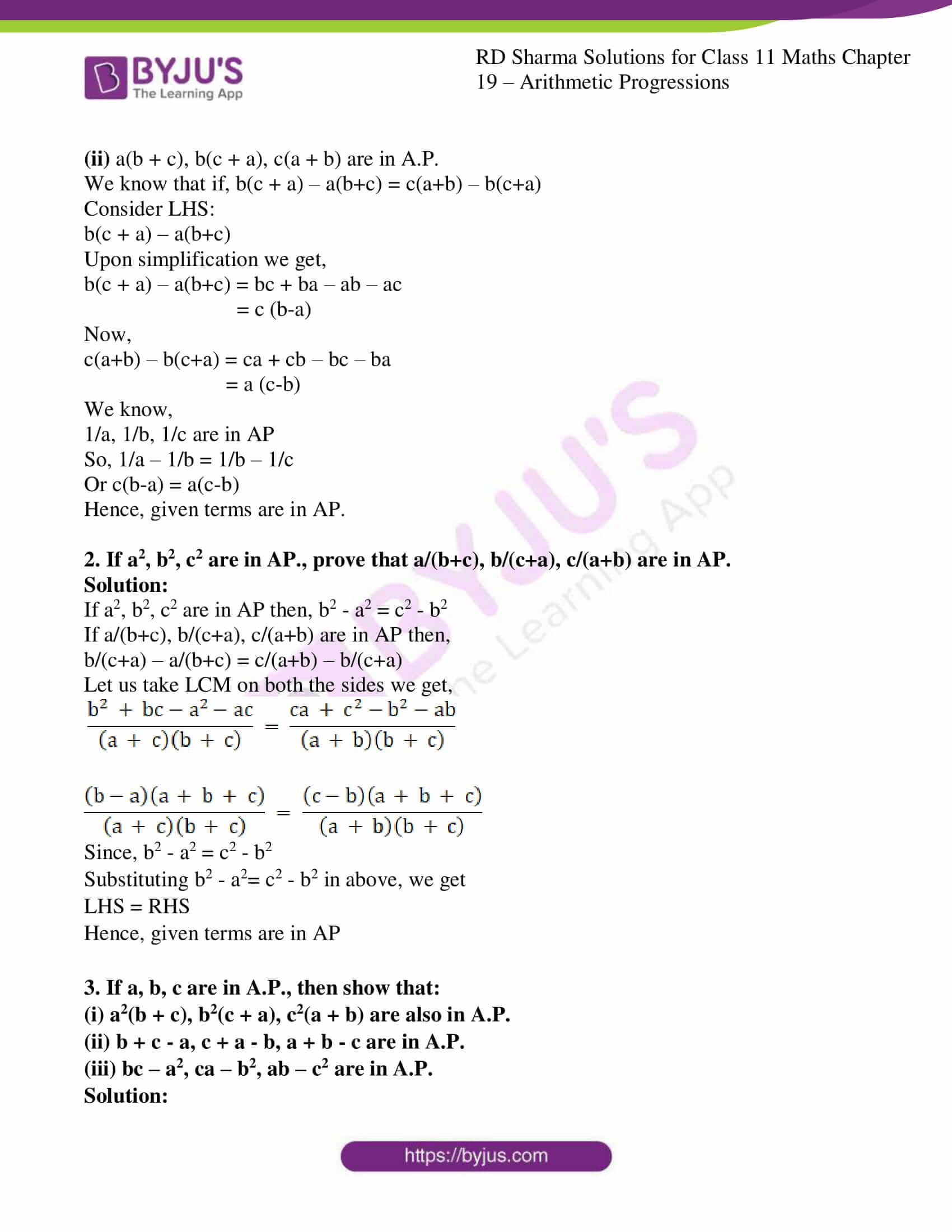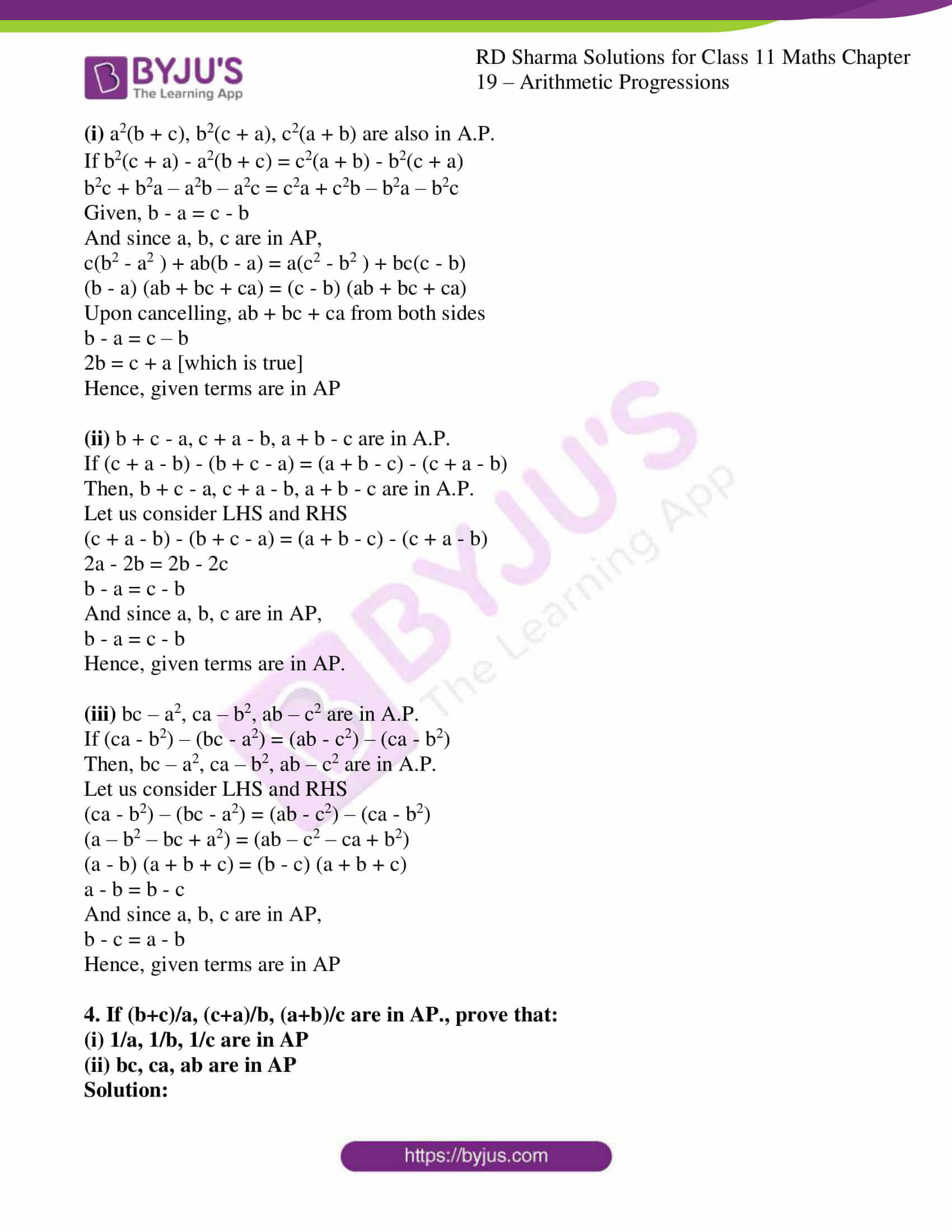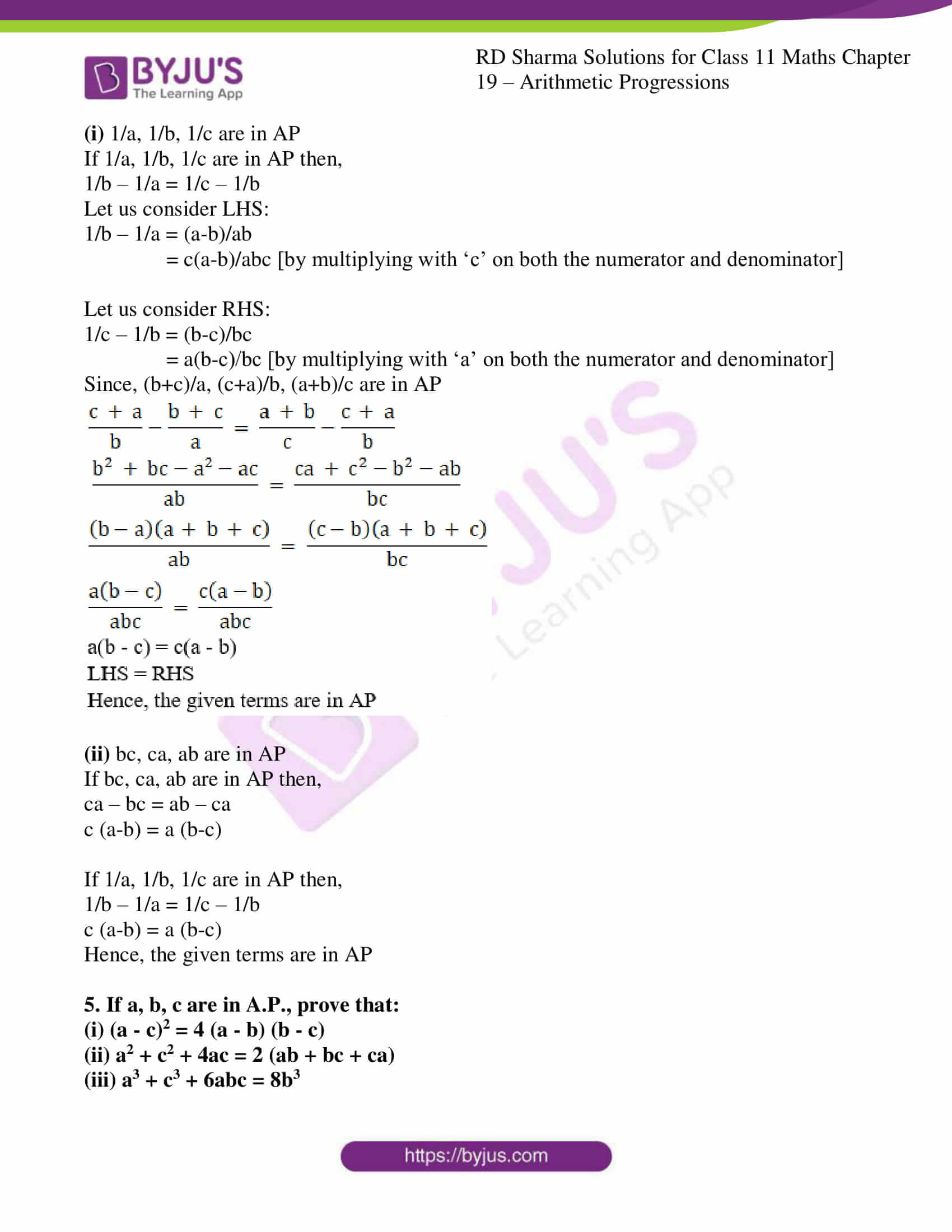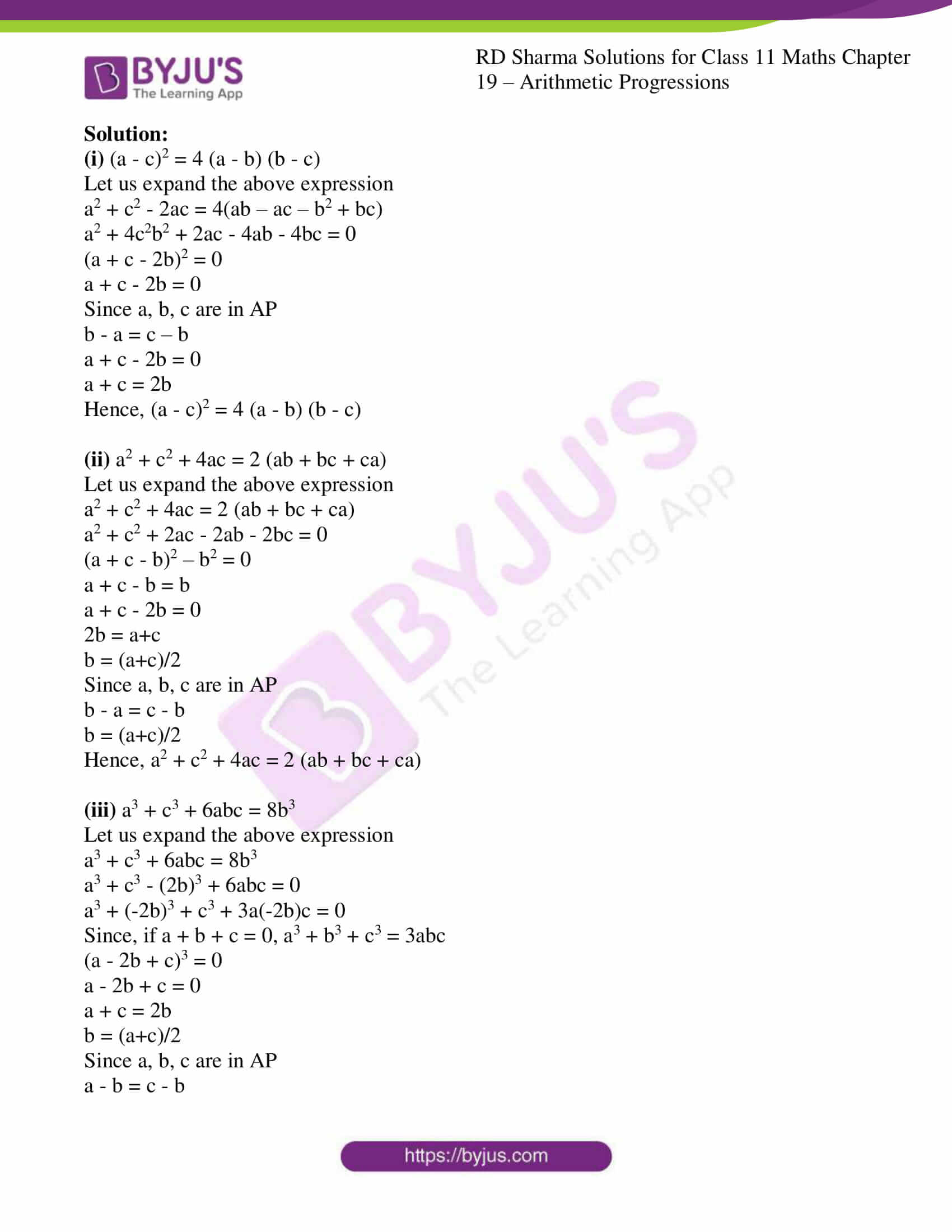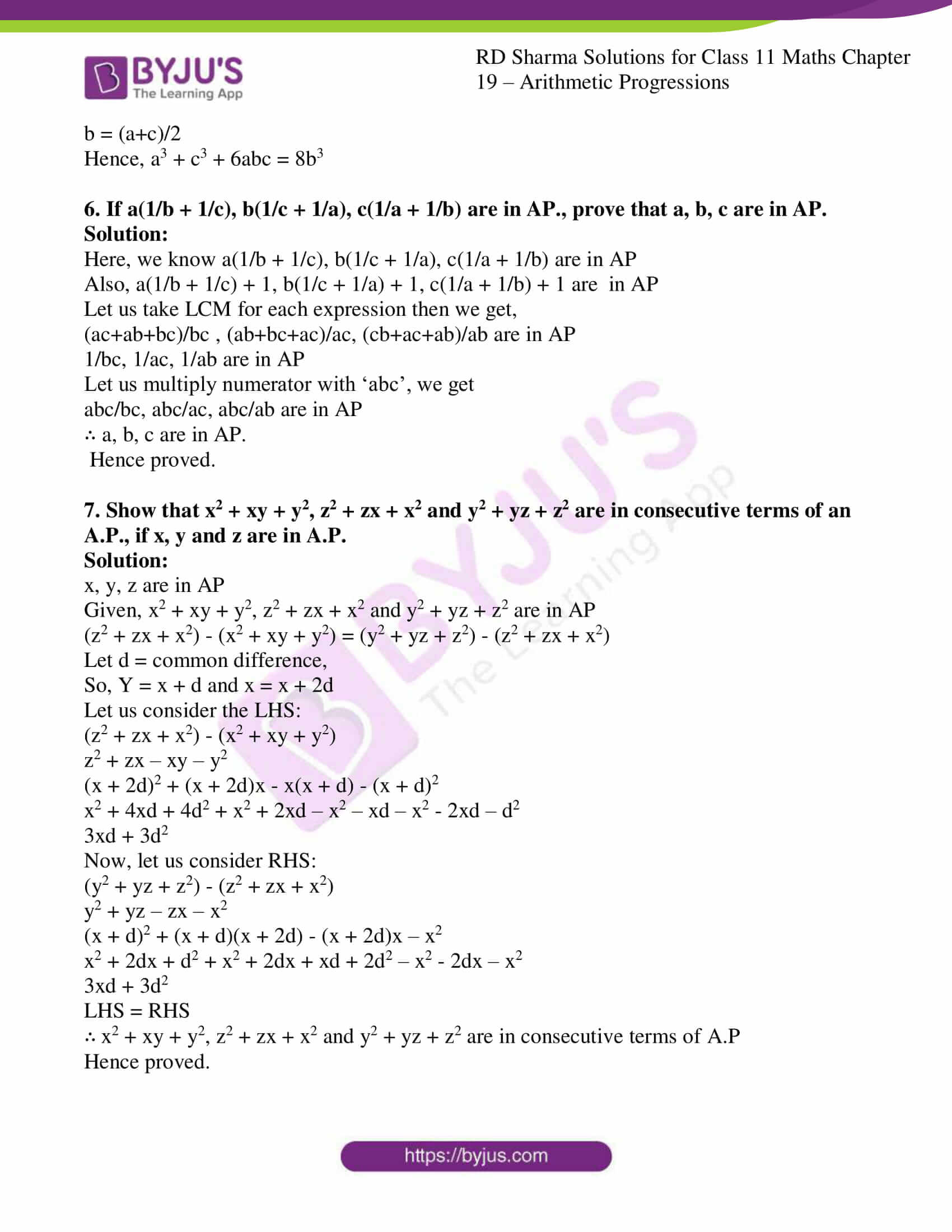### Also, access other exercises of RD Sharma Solutions for Class 11 Maths Chapter 19 – Arithmetic Progressions

Exercise 19.1 Solutions

Exercise 19.2 Solutions

Exercise 19.3 Solutions

Exercise 19.4 Solutions

Exercise 19.6 Solutions

Exercise 19.7 Solutions

### Access answers to RD Sharma Solutions for Class 11 Maths Exercise 19.5 Chapter 19 – Arithmetic Progressions

1. If 1/a, 1/b, 1/c are in A.P., prove that:

(i) (b+c)/a, (c+a)/b, (a+b)/c are in A.P.

(ii) a(b + c), b(c + a), c(a + b) are in A.P.

Solution:

(i) (b+c)/a, (c+a)/b, (a+b)/c are in A.P.

We know that, if a, b, c are in AP, then b – a = c – b

If, 1/a, 1/b, 1/c are in AP

Then, 1/b – 1/a = 1/c – 1/b

If (b+c)/a, (c+a)/b, (a+b)/c are in AP

Then, (c+a)/b – (b+c)/a = (a+b)/c – (c+a)/b

Let us take LCM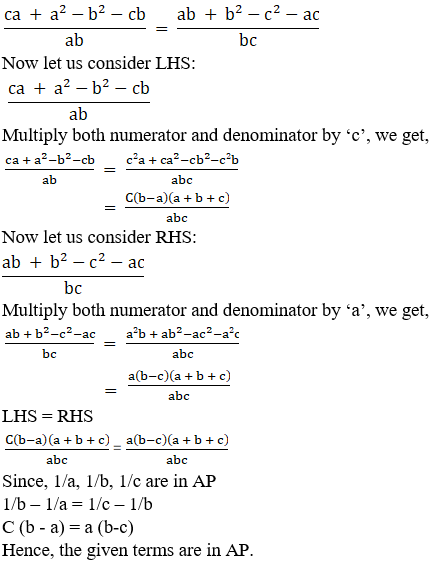Since, 1/a, 1/b, 1/c are in AP

1/b – 1/a = 1/c – 1/b

C (b – a) = a (b-c)

Hence, the given terms are in AP.

(ii) a(b + c), b(c + a), c(a + b) are in A.P.

We know that if, b(c + a) – a(b+c) = c(a+b) – b(c+a)

Consider LHS:

b(c + a) – a(b+c)

Upon simplification we get,

b(c + a) – a(b+c) = bc + ba – ab – ac

= c (b-a)

Now,

c(a+b) – b(c+a) = ca + cb – bc – ba

= a (c-b)

We know,

1/a, 1/b, 1/c are in AP

So, 1/a – 1/b = 1/b – 1/c

Or c(b-a) = a(c-b)

Hence, given terms are in AP.

2. If a2, b2, c2 are in AP., prove that a/(b+c), b/(c+a), c/(a+b) are in AP.

Solution:

If a2, b2, c2 are in AP then, b2 – a2 = c2 – b2

If a/(b+c), b/(c+a), c/(a+b) are in AP then,

b/(c+a) – a/(b+c) = c/(a+b) – b/(c+a)

Let us take LCM on both the sides we get,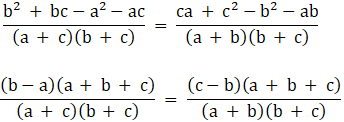Since, b2 – a2 = c2 – b2

Substituting b2 – a2= c2 – b2 in above, we get

LHS = RHS

Hence, given terms are in AP

3. If a, b, c are in A.P., then show that:
(i) a2(b + c), b2(c + a), c2(a + b) are also in A.P.
(ii) b + c – a, c + a – b, a + b – c are in A.P.
(iii) bc – a2, ca – b2, ab – c2 are in A.P.

Solution:

(i) a2(b + c), b2(c + a), c2(a + b) are also in A.P.

If b2(c + a) – a2(b + c) = c2(a + b) – b2(c + a)

b2c + b2a – a2b – a2c = c2a + c2b – b2a – b2c

Given, b – a = c – b

And since a, b, c are in AP,

c(b2 – a2 ) + ab(b – a) = a(c2 – b2 ) + bc(c – b)

(b – a) (ab + bc + ca) = (c – b) (ab + bc + ca)

Upon cancelling, ab + bc + ca from both sides

b – a = c – b

2b = c + a [which is true]

Hence, given terms are in AP

(ii) b + c – a, c + a – b, a + b – c are in A.P.

If (c + a – b) – (b + c – a) = (a + b – c) – (c + a – b)

Then, b + c – a, c + a – b, a + b – c are in A.P.

Let us consider LHS and RHS

(c + a – b) – (b + c – a) = (a + b – c) – (c + a – b)

2a – 2b = 2b – 2c

b – a = c – b

And since a, b, c are in AP,

b – a = c – b

Hence, given terms are in AP.

(iii) bc – a2, ca – b2, ab – c2 are in A.P.

If (ca – b2) – (bc – a2) = (ab – c2) – (ca – b2)

Then, bc – a2, ca – b2, ab – c2 are in A.P.

Let us consider LHS and RHS

(ca – b2) – (bc – a2) = (ab – c2) – (ca – b2)

(a – b2 – bc + a2) = (ab – c2 – ca + b2)

(a – b) (a + b + c) = (b – c) (a + b + c)

a – b = b – c

And since a, b, c are in AP,

b – c = a – b

Hence, given terms are in AP

4. If (b+c)/a, (c+a)/b, (a+b)/c are in AP., prove that:

(i) 1/a, 1/b, 1/c are in AP

(ii) bc, ca, ab are in AP

Solution:

(i) 1/a, 1/b, 1/c are in AP

If 1/a, 1/b, 1/c are in AP then,

1/b – 1/a = 1/c – 1/b

Let us consider LHS:

1/b – 1/a = (a-b)/ab

= c(a-b)/abc [by multiplying with ‘c’ on both the numerator and denominator]

Let us consider RHS:

1/c – 1/b = (b-c)/bc

= a(b-c)/bc [by multiplying with ‘a’ on both the numerator and denominator]

Since, (b+c)/a, (c+a)/b, (a+b)/c are in AP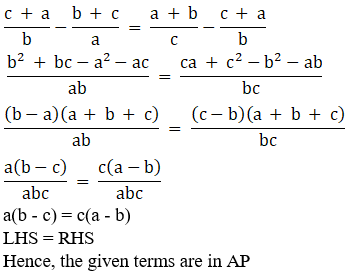(ii) bc, ca, ab are in AP

If bc, ca, ab are in AP then,

ca – bc = ab – ca

c (a-b) = a (b-c)

If 1/a, 1/b, 1/c are in AP then,

1/b – 1/a = 1/c – 1/b

c (a-b) = a (b-c)

Hence, the given terms are in AP

5. If a, b, c are in A.P., prove that:
(i) (a – c)2 = 4 (a – b) (b – c)

(ii) a2 + c2 + 4ac = 2 (ab + bc + ca)

(iii) a3 + c3 + 6abc = 8b3

Solution:

(i) (a – c)2 = 4 (a – b) (b – c)

Let us expand the above expression

a2 + c2 – 2ac = 4(ab – ac – b2 + bc)

a2 + 4c2b2 + 2ac – 4ab – 4bc = 0

(a + c – 2b)2 = 0

a + c – 2b = 0

Since a, b, c are in AP

b – a = c – b

a + c – 2b = 0

a + c = 2b

Hence, (a – c)2 = 4 (a – b) (b – c)

(ii) a2 + c2 + 4ac = 2 (ab + bc + ca)

Let us expand the above expression

a2 + c2 + 4ac = 2 (ab + bc + ca)

a2 + c2 + 2ac – 2ab – 2bc = 0

(a + c – b)2 – b2 = 0

a + c – b = b

a + c – 2b = 0

2b = a+c

b = (a+c)/2

Since a, b, c are in AP

b – a = c – b

b = (a+c)/2

Hence, a2 + c2 + 4ac = 2 (ab + bc + ca)

(iii) a3 + c3 + 6abc = 8b3

Let us expand the above expression

a3 + c3 + 6abc = 8b3

a3 + c3 – (2b)3 + 6abc = 0

a3 + (-2b)3 + c3 + 3a(-2b)c = 0

Since, if a + b + c = 0, a3 + b3 + c3 = 3abc

(a – 2b + c)3 = 0

a – 2b + c = 0

a + c = 2b

b = (a+c)/2

Since a, b, c are in AP

a – b = c – b

b = (a+c)/2

Hence, a3 + c3 + 6abc = 8b3

6. If a(1/b + 1/c), b(1/c + 1/a), c(1/a + 1/b) are in AP., prove that a, b, c are in AP.

Solution:

Here, we know a(1/b + 1/c), b(1/c + 1/a), c(1/a + 1/b) are in AP

Also, a(1/b + 1/c) + 1, b(1/c + 1/a) + 1, c(1/a + 1/b) + 1 are in AP

Let us take LCM for each expression then we get,

(ac+ab+bc)/bc , (ab+bc+ac)/ac, (cb+ac+ab)/ab are in AP

1/bc, 1/ac, 1/ab are in AP

Let us multiply numerator with ‘abc’, we get

abc/bc, abc/ac, abc/ab are in AP

∴ a, b, c are in AP.

Hence proved.

7. Show that x2 + xy + y2, z2 + zx + x2 and y2 + yz + z2 are in consecutive terms of an A.P., if x, y and z are in A.P.

Solution:

x, y, z are in AP

Given, x2 + xy + y2, z2 + zx + x2 and y2 + yz + z2 are in AP

(z2 + zx + x2) – (x2 + xy + y2) = (y2 + yz + z2) – (z2 + zx + x2)

Let d = common difference,

So, Y = x + d and x = x + 2d

Let us consider the LHS:

(z2 + zx + x2) – (x2 + xy + y2)

z2 + zx – xy – y2

(x + 2d)2 + (x + 2d)x – x(x + d) – (x + d)2

x2 + 4xd + 4d2 + x2 + 2xd – x2 – xd – x2 – 2xd – d2

3xd + 3d2

Now, let us consider RHS:

(y2 + yz + z2) – (z2 + zx + x2)

y2 + yz – zx – x2

(x + d)2 + (x + d)(x + 2d) – (x + 2d)x – x2

x2 + 2dx + d2 + x2 + 2dx + xd + 2d2 – x2 – 2dx – x2

3xd + 3d2

LHS = RHS

∴ x2 + xy + y2, z2 + zx + x2 and y2 + yz + z2 are in consecutive terms of A.P

Hence proved.